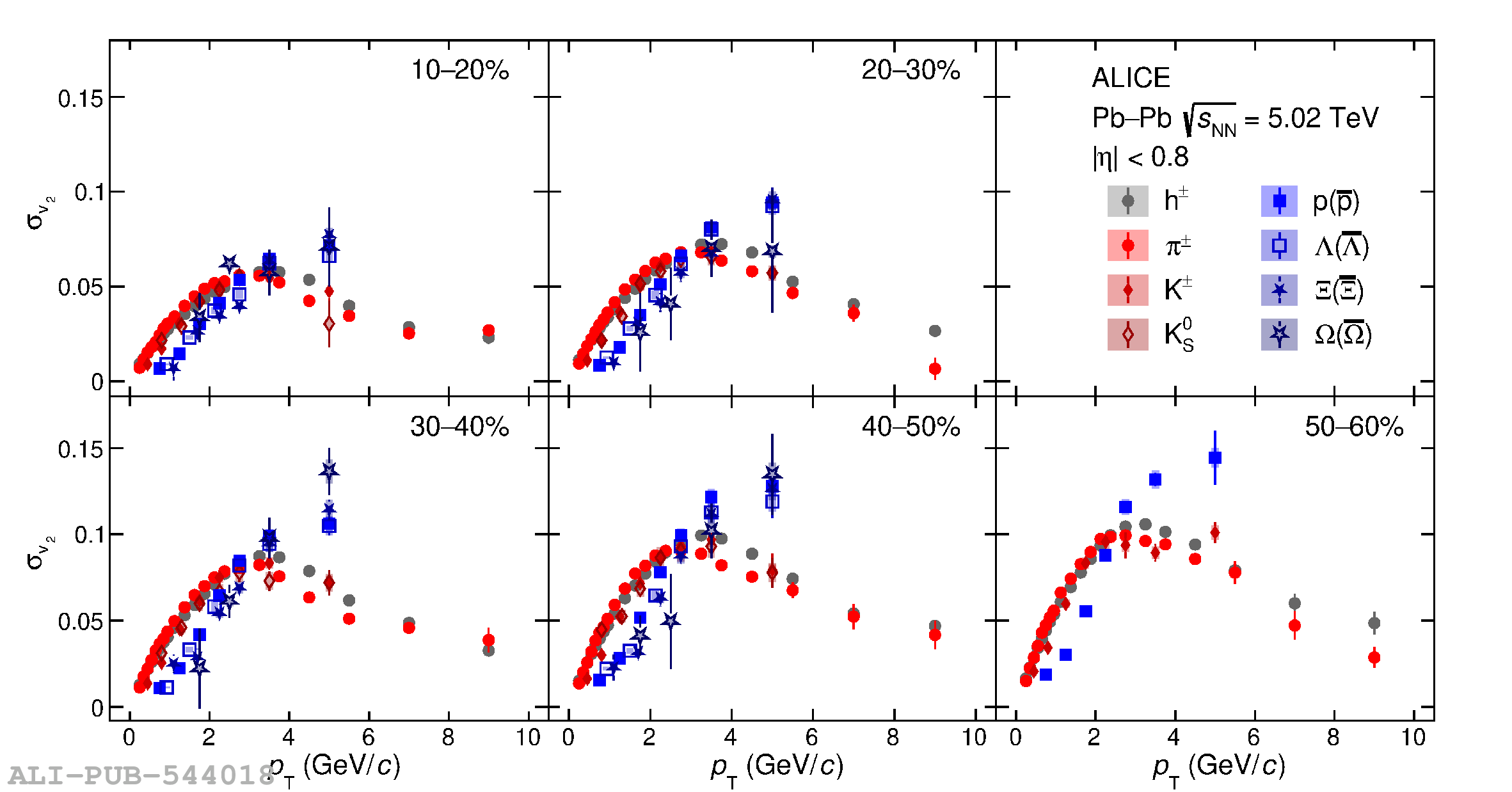# Figure 6

 The \pt{} dependence of the standard deviation of $v_2$ ($\sigma_{\rm v_2}$) for different particle species and centralities in Pb--Pb collisions at $\sqrt{s_{\mathrm{NN}}} = 5.02$ TeV. The vertical error bars and the filled boxes represent statistical and systematic uncertainties, respectively.